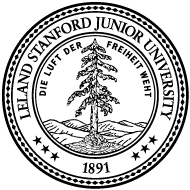# Seminar on the Foundations of Mathematics

### Date : Monday 4 - 5:30pmThe focus of this seminar will be to take a careful look at many mathematical ideas and concepts that we deem to be "simple" or "obvious". Things like "what is a number?", "what is a proof?", "what is a set?", "what is a random variable?" etc.

We'll spend time focusing on "why" questions. In particular, we'll ask questions like why certain schools of reasoning define or assume (as "true") things that fail to make sense in the context of realization on mathematical machines (aka. computing machines that we can physically construct).

On a more technical note, the seminar aims to expose participants to ideas from Philosophy ("What is a Proof?"), Logic, Mathematical Languages, Arithmetic, Type Theory, Homotopy Type Theory (univalent foundations), Quantum computation (and randomness), Games, and Machine Computation. The topics are vast, and so we'll cherry pick things that are of interest to folks involved.

### July 28, 2017: Control

#### Presented by : Samir Menon

This session will discuss early efforts in developing control theory within the context of robotics and dynamics. The various attempts to connect conceptual desired state in arbitrary spaces to actuator spaces for physical systems. We discuss outstanding problems at the heart of control theory, related to the digital computation vs. analog physical system divide. Motivate efforts in mathematics for developing novel mathematical formulations that go beyond numerical methods in addressing this divide.

### July 06, 2017: Arithmetic

#### Presented by : Samir Menon

This session will discuss Frege's attempts to provide a foundation of the notion of number and, as such, arithmetic. There are a few very interesting arguments related to the zero, the minimum specification required for an arithmetic rule, and rule iteration. A few complex arguments are made to ground the idea of number in thought. It is evident that Frege thought deeply about even simple ideas; it can provide some insight into his (likely) criticism of Hilbert's work.

### July 03, 2017: Probability

#### Presented by : Ozan Sener and Shameek Ganguly

This session will discuss past attempts to construct a theory of probability, and different views of randomness. To note a few interesting topics. What are the different schools of probabilistic thought? Frequentist vs. Bayesian ideas. Kolmogorov's probability. Intuitionist probability. Games against nature. Random sequences.

We will also discuss what definitions of probability work well with finite memory and finite time computing systems? This should include a discussion of random number generators, translation and scale invariance of the probability measure space, quantum computing etc.

### June 26, 2017: Proof

#### Presented by : Samir Menon and Vikranth Reddy

Lakatos offers a view of the idea of "proof" as one that involves the articulation of an idea in a manner that admits further inquiry. He then proceeds to demonstrate that the statement of a proof often inaccurately captures the idea. As a consequence, it is feasible to "refute" the proof with counter examples in said language. He then discusses types of refutations, and develops a general theory of how to use refutations to improve "proofs" in a systematic manner. The crux of his argument is that refutations are just as important as proofs, and that they are an integral part of the process of clearly articulating an idea.

Terry Tao says something quite similar in this blog post.